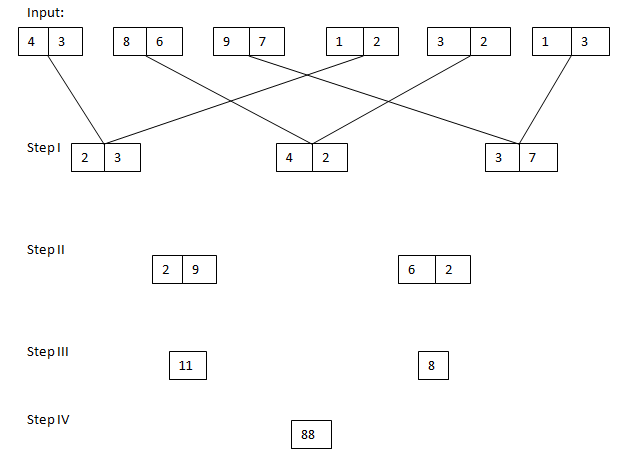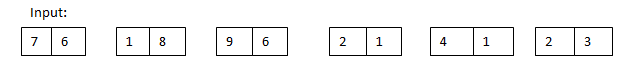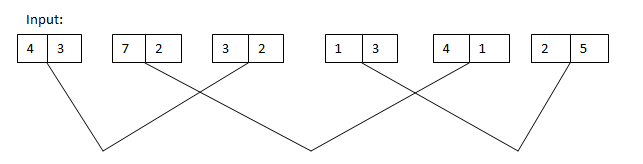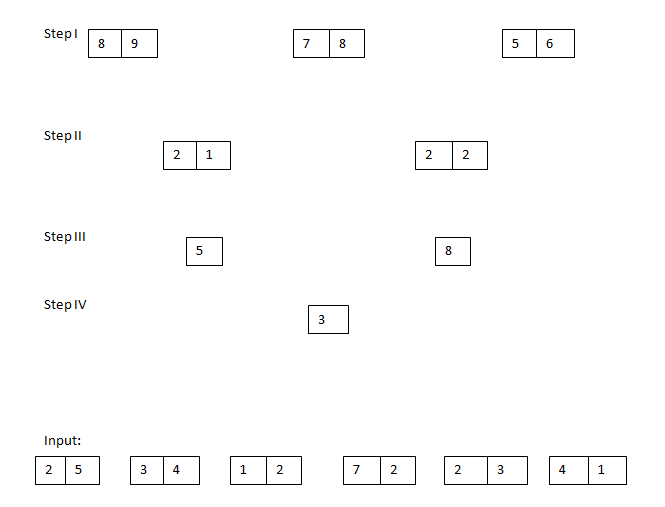# Important Questions BASED on NEW Pattern (Day-21): Reasoning(Input Output):

Important Questions BASED on NEW Pattern (Day-21): Reasoning(Input output):

Dear Readers, IBPS Examinations was approaching shortly and many of our followers were requesting us to provide Practice Questions based on the new pattern, for that here WE have started providing Practice Questions Based on New pattern from Aptitude, Reasoning and English each one topic daily. Kindly follow us regularly and make use of it, if you have any suggestion kindly use the comment section below.

[WpProQuiz 571]

Directions (1-5): Study the following information carefully and answer the given questions.

A number arrangement machine arranges two digit numbers into a typical manner. Each step gives output taking input from the previous step. The following is an illustration of Input and rearrangement. Using the illustration answer the question given below.1.Which of the following represent the difference between the first digit of the second number and second digit of the first number in step II?

a) 7

b) 9

c) 5

d) 3

e) None of these

1. If each digit in the step II is subtracted by value “2” and then added all digits, then what will be the final sum?

a) 12

b) 17

c) 10

d) 19

e) None of these

3.What is the difference of two numbers obtained in step III?

a) 8

b) 10

c) 12

d) 16

e) None of these

4.If the value ‘6’ is subtracted from the final output, then what will be the resultant value?

a) 42

b) 35

c) 64

d) 50

e) None of these

5.Which of the following combination represent the first digit of the third number and second digit of the first number in step I of the given input?

a) 2, 7

b) 3, 2

c) 1, 7

d) 2, 1

e) 3, 3

Directions (6-10): Study the following information carefully and answer the given questions.

A number arrangement machine arranges two digit numbers into a typical manner. Each step gives output taking input from the previous step. The following is an illustration of Input and rearrangement. Using the illustration answer the question given below.6.Which of the following combination represent the first digit of the third number and second digit of the first number in step I of the given input?

a) 8, 9

b) 8, 4

c) 7, 4

d) 7, 5

e) 9, 4

7.What is the multiplication of two numbers obtained in step III?

a) 724

b) 656

c) 570

d) 818

e) None of these

1. If each digit in the step II is squared and then added, then what will be the final sum?

a) 82

b) 96

c) 74

d) 85

e) 90

9.If the value ‘8^2’ is subtracted from the final output, then what will be the resultant value?

a) 8

b) 12

c) 10

d) 24

e) None of these

10.Which of the following represent the difference between the second digit of the second number and first digit of the first number in step II?

a) 1

b) 0

c) 2

d) 3

e) None of these

[WpProQuiz 571]